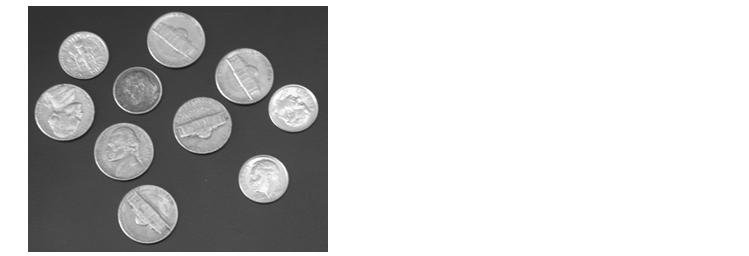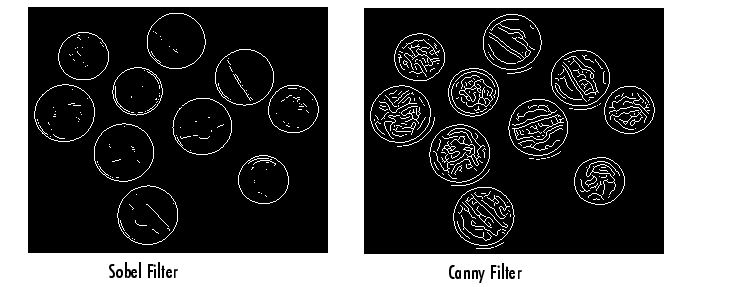Image Processing Toolbox User's GuideAnalyzing an Image

Image analysis techniques return information about the structure of an image. This section describes toolbox functions that you can use for these image analysis techniques:

The toolbox also includes functions that return information about the texture of an image. See Analyzing the Texture of an Image for more information.

Detecting Edges

In an image, an edge is a curve that follows a path of rapid change in image intensity. Edges are often associated with the boundaries of objects in a scene. Edge detection is used to identify the edges in an image.

To find edges, you can use the `edge` function. This function looks for places in the image where the intensity changes rapidly, using one of these two criteria:

• Places where the first derivative of the intensity is larger in magnitude than some threshold
• Places where the second derivative of the intensity has a zero crossing

`edge` provides a number of derivative estimators, each of which implements one of the definitions above. For some of these estimators, you can specify whether the operation should be sensitive to horizontal edges, vertical edges, or both. `edge` returns a binary image containing 1's where edges are found and 0's elsewhere.

The most powerful edge-detection method that `edge` provides is the Canny method. The Canny method differs from the other edge-detection methods in that it uses two different thresholds (to detect strong and weak edges), and includes the weak edges in the output only if they are connected to strong edges. This method is therefore less likely than the others to be fooled by noise, and more likely to detect true weak edges.

The following example illustrates the power of the Canny edge detector by showing the results of applying the Sobel and Canny edge detectors to the same image:

1. Read image and display it.
• ```I = imread('coins.png');
imshow(I)```
2. Apply the Sobel and Canny edge detectors to the image and display them.
• ```BW1 = edge(I,'sobel');
BW2 = edge(I,'canny');
imshow(BW1)
figure, imshow(BW2)```

For an interactive demonstration of edge detection, try running `edgedemo`.Computing Properties for Image Regions Tracing Boundaries